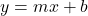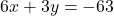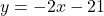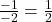What is the slope of a line perpendicular to the line whose equation is 6x+3y=-63

Question

What is the slope of a line perpendicular to the line whose equation is 6x+3y=-63

in progress 0
5 months 2021-08-15T07:51:03+00:00 2 Answers 21 views 0

y=x/2−63

Step-by-step explanation:  6x+3y=-63     3y=6x-63= y=2/x-63

The slope of a line perpendicular to the line whose equation is 6x+3y=-63 will be: 1/2

Step-by-step explanation:

We know that the slope intercept-form of the line equation iswhere m is the slope and b is the y-intercept

Given the equationsimplifying the equation to write in the slope-intercept formThus, the slope = -2

As we know that the slope of the perpendicular line is basically the negative reciprocal of the slope of the line, so

The slope of the perpendicular line will be:Therefore, the slope of a line perpendicular to the line whose equation is 6x+3y=-63 will be: 1/2